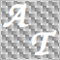• Übersicht
• Bewertungen (2)
• Diskussion (2)

# FT ROC Histogram MT4

Indicator that plots the ROC (Rate of change) values from the selected price or moving average as a histogram with smoothing line.

### Displayed Data

• ExtROC - Rate of change.
• ExtMAROC - Smoothing line.

### Inputs

• InpCalculation - You can choose between price and moving average. From this values will be calculated ROC.
• InpCalculationPriceAppliedPrice - Applied price when calculating with price (Close, High, Low, Median, Open, Typical, Weighted).
• InpCalculationMAPeriod - Moving average period when calculating with moving average.
• InpCalculationMAAppliedPrice - Moving average applied price when calculating with moving average (Close, High, Low, Median, Open, Typical, Weighted).
• InpCalculationMAMethod - Moving average method when calculating with moving average (SMA, EMA, LWMA, SMMA).
• InpROCPeriod - Rate of change period.
• InpMAROCMethod - Moving average method for smoothing (SMA, EMA, LWMA, SMMA).
• InpMAROCPeriod - Moving average period for smoothing.438
2017.02.22 21:09

Thanks for this usefull indi !! Equilibrium of Price is easier to find and percentage pricechange is never to ignore now.21351
2016.08.31 15:32

Der Benutzer hat keinen Kommentar hinterlassen